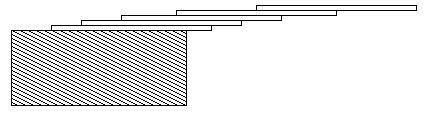# poj 1003:Hangover（水题，数学模拟）

Hangover
 Time Limit: 1000MS Memory Limit: 10000K Total Submissions: 99450 Accepted: 48213

Description

How far can you make a stack of cards overhang a table? If you have one card, you can create a maximum overhang of half a card length. (We're assuming that the cards must be perpendicular to the table.) With two cards you can make the top card overhang the bottom one by half a card length, and the bottom one overhang the table by a third of a card length, for a total maximum overhang of 1/2 + 1/3 = 5/6 card lengths. In general you can make n cards overhang by 1/2 + 1/3 + 1/4 + ... + 1/(n + 1) card lengths, where the top card overhangs the second by 1/2, the second overhangs tha third by 1/3, the third overhangs the fourth by 1/4, etc., and the bottom card overhangs the table by 1/(n + 1). This is illustrated in the figure below.Input

The input consists of one or more test cases, followed by a line containing the number 0.00 that signals the end of the input. Each test case is a single line containing a positive floating-point number c whose value is at least 0.01 and at most 5.20; c will contain exactly three digits.

Output

For each test case, output the minimum number of cards necessary to achieve an overhang of at least c card lengths. Use the exact output format shown in the examples.

Sample Input

```1.00
3.71
0.04
5.19
0.00
```

Sample Output

```3 card(s)
61 card(s)
1 card(s)
273 card(s)```

Source

水题，模拟
题意求最小的n让1+1/2+1/3+...+1/n大于给的一个实数。
思路：找一个数sum记录结果，写一个循环不断累加 sum+=1.0/n，直到sum大于c，输出n-2.
代码：
``` 1 #include <iostream>
2
3 using namespace std;
4
5 int main()
6 {
7     double c,sum;
8     int n,card;
9     while(cin>>c){
10         if(int(c*100)==0) break;
11         sum = 0;
12         n=2;
13         while(sum<=c){  //循环模拟。直到超过
14             sum+=1.0/n;
15             n++;
16         }
17         cout<<n-2<<" card(s)"<<endl;
18     }
19     return 0;
20 }```

Freecode : www.cnblogs.com/yym2013

##原创声明 **转载请注明：[呓语](http://www.yangyingming.com) » [poj 1003:Hangover（水题，数学模拟）](http://www.yangyingming.com/article/90)**